Docs for 0.15.0
All versions

# Module: morphology¶

 skimage.morphology.binary_erosion(image[, …]) Return fast binary morphological erosion of an image. skimage.morphology.binary_dilation(image[, …]) Return fast binary morphological dilation of an image. skimage.morphology.binary_opening(image[, …]) Return fast binary morphological opening of an image. skimage.morphology.binary_closing(image[, …]) Return fast binary morphological closing of an image. skimage.morphology.erosion(image[, selem, …]) Return greyscale morphological erosion of an image. skimage.morphology.dilation(image[, selem, …]) Return greyscale morphological dilation of an image. skimage.morphology.opening(image[, selem, out]) Return greyscale morphological opening of an image. skimage.morphology.closing(image[, selem, out]) Return greyscale morphological closing of an image. skimage.morphology.white_tophat(image[, …]) Return white top hat of an image. skimage.morphology.black_tophat(image[, …]) Return black top hat of an image. skimage.morphology.square(width[, dtype]) Generates a flat, square-shaped structuring element. skimage.morphology.rectangle(width, height) Generates a flat, rectangular-shaped structuring element. skimage.morphology.diamond(radius[, dtype]) Generates a flat, diamond-shaped structuring element. skimage.morphology.disk(radius[, dtype]) Generates a flat, disk-shaped structuring element. skimage.morphology.cube(width[, dtype]) Generates a cube-shaped structuring element. skimage.morphology.octahedron(radius[, dtype]) Generates a octahedron-shaped structuring element. skimage.morphology.ball(radius[, dtype]) Generates a ball-shaped structuring element. skimage.morphology.octagon(m, n[, dtype]) Generates an octagon shaped structuring element. skimage.morphology.star(a[, dtype]) Generates a star shaped structuring element. skimage.morphology.label(input[, neighbors, …]) Label connected regions of an integer array. skimage.morphology.watershed(image[, …]) Find watershed basins in image flooded from given markers. skimage.morphology.skeletonize(image) Return the skeleton of a binary image. skimage.morphology.skeletonize_3d(img) Compute the skeleton of a binary image. skimage.morphology.thin(image[, max_iter]) Perform morphological thinning of a binary image. skimage.morphology.medial_axis(image[, …]) Compute the medial axis transform of a binary image skimage.morphology.convex_hull_image(image) Compute the convex hull image of a binary image. skimage.morphology.convex_hull_object(image) Compute the convex hull image of individual objects in a binary image. skimage.morphology.reconstruction(seed, mask) Perform a morphological reconstruction of an image. skimage.morphology.remove_small_objects(ar) Remove objects smaller than the specified size. skimage.morphology.remove_small_holes(ar[, …]) Remove contiguous holes smaller than the specified size. skimage.morphology.h_minima(image, h[, selem]) Determine all minima of the image with depth >= h. skimage.morphology.h_maxima(image, h[, selem]) Determine all maxima of the image with height >= h. skimage.morphology.local_maxima(image[, …]) Find local maxima of n-dimensional array. skimage.morphology.local_minima(image[, …]) Find local minima of n-dimensional array. skimage.morphology.flood(image, seed_point, *) Mask corresponding to a flood fill. skimage.morphology.flood_fill(image, …[, …]) Perform flood filling on an image. skimage.morphology.max_tree(image[, …]) Build the max tree from an image. skimage.morphology.area_opening(image[, …]) Perform an area opening of the image. skimage.morphology.area_closing(image[, …]) Perform an area closing of the image. skimage.morphology.diameter_opening(image[, …]) Perform a diameter opening of the image. skimage.morphology.diameter_closing(image[, …]) Perform a diameter closing of the image. skimage.morphology.max_tree_local_maxima(image) Determine all local maxima of the image.

## binary_erosion¶

skimage.morphology.binary_erosion(image, selem=None, out=None)[source]

Return fast binary morphological erosion of an image.

This function returns the same result as greyscale erosion but performs faster for binary images.

Morphological erosion sets a pixel at (i,j) to the minimum over all pixels in the neighborhood centered at (i,j). Erosion shrinks bright regions and enlarges dark regions.

Parameters: image : ndarray Binary input image. selem : ndarray, optional The neighborhood expressed as a 2-D array of 1’s and 0’s. If None, use cross-shaped structuring element (connectivity=1). out : ndarray of bool, optional The array to store the result of the morphology. If None is passed, a new array will be allocated. eroded : ndarray of bool or uint The result of the morphological erosion taking values in [False, True].

## binary_dilation¶

skimage.morphology.binary_dilation(image, selem=None, out=None)[source]

Return fast binary morphological dilation of an image.

This function returns the same result as greyscale dilation but performs faster for binary images.

Morphological dilation sets a pixel at (i,j) to the maximum over all pixels in the neighborhood centered at (i,j). Dilation enlarges bright regions and shrinks dark regions.

Parameters: image : ndarray Binary input image. selem : ndarray, optional The neighborhood expressed as a 2-D array of 1’s and 0’s. If None, use cross-shaped structuring element (connectivity=1). out : ndarray of bool, optional The array to store the result of the morphology. If None, is passed, a new array will be allocated. dilated : ndarray of bool or uint The result of the morphological dilation with values in [False, True].

## binary_opening¶

skimage.morphology.binary_opening(image, selem=None, out=None)[source]

Return fast binary morphological opening of an image.

This function returns the same result as greyscale opening but performs faster for binary images.

The morphological opening on an image is defined as an erosion followed by a dilation. Opening can remove small bright spots (i.e. “salt”) and connect small dark cracks. This tends to “open” up (dark) gaps between (bright) features.

Parameters: image : ndarray Binary input image. selem : ndarray, optional The neighborhood expressed as a 2-D array of 1’s and 0’s. If None, use cross-shaped structuring element (connectivity=1). out : ndarray of bool, optional The array to store the result of the morphology. If None is passed, a new array will be allocated. opening : ndarray of bool The result of the morphological opening.

## binary_closing¶

skimage.morphology.binary_closing(image, selem=None, out=None)[source]

Return fast binary morphological closing of an image.

This function returns the same result as greyscale closing but performs faster for binary images.

The morphological closing on an image is defined as a dilation followed by an erosion. Closing can remove small dark spots (i.e. “pepper”) and connect small bright cracks. This tends to “close” up (dark) gaps between (bright) features.

Parameters: image : ndarray Binary input image. selem : ndarray, optional The neighborhood expressed as a 2-D array of 1’s and 0’s. If None, use cross-shaped structuring element (connectivity=1). out : ndarray of bool, optional The array to store the result of the morphology. If None, is passed, a new array will be allocated. closing : ndarray of bool The result of the morphological closing.

## erosion¶

skimage.morphology.erosion(image, selem=None, out=None, shift_x=False, shift_y=False)[source]

Return greyscale morphological erosion of an image.

Morphological erosion sets a pixel at (i,j) to the minimum over all pixels in the neighborhood centered at (i,j). Erosion shrinks bright regions and enlarges dark regions.

Parameters: image : ndarray Image array. selem : ndarray, optional The neighborhood expressed as an array of 1’s and 0’s. If None, use cross-shaped structuring element (connectivity=1). out : ndarrays, optional The array to store the result of the morphology. If None is passed, a new array will be allocated. shift_x, shift_y : bool, optional shift structuring element about center point. This only affects eccentric structuring elements (i.e. selem with even numbered sides). eroded : array, same shape as image The result of the morphological erosion.

Notes

For uint8 (and uint16 up to a certain bit-depth) data, the lower algorithm complexity makes the skimage.filters.rank.minimum function more efficient for larger images and structuring elements.

Examples

>>> # Erosion shrinks bright regions
>>> import numpy as np
>>> from skimage.morphology import square
>>> bright_square = np.array([[0, 0, 0, 0, 0],
...                           [0, 1, 1, 1, 0],
...                           [0, 1, 1, 1, 0],
...                           [0, 1, 1, 1, 0],
...                           [0, 0, 0, 0, 0]], dtype=np.uint8)
>>> erosion(bright_square, square(3))
array([[0, 0, 0, 0, 0],
[0, 0, 0, 0, 0],
[0, 0, 1, 0, 0],
[0, 0, 0, 0, 0],
[0, 0, 0, 0, 0]], dtype=uint8)


### Examples using skimage.morphology.erosion¶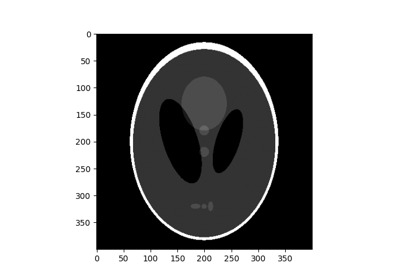Morphological Filtering

## dilation¶

skimage.morphology.dilation(image, selem=None, out=None, shift_x=False, shift_y=False)[source]

Return greyscale morphological dilation of an image.

Morphological dilation sets a pixel at (i,j) to the maximum over all pixels in the neighborhood centered at (i,j). Dilation enlarges bright regions and shrinks dark regions.

Parameters: image : ndarray Image array. selem : ndarray, optional The neighborhood expressed as a 2-D array of 1’s and 0’s. If None, use cross-shaped structuring element (connectivity=1). out : ndarray, optional The array to store the result of the morphology. If None, is passed, a new array will be allocated. shift_x, shift_y : bool, optional shift structuring element about center point. This only affects eccentric structuring elements (i.e. selem with even numbered sides). dilated : uint8 array, same shape and type as image The result of the morphological dilation.

Notes

For uint8 (and uint16 up to a certain bit-depth) data, the lower algorithm complexity makes the skimage.filters.rank.maximum function more efficient for larger images and structuring elements.

Examples

>>> # Dilation enlarges bright regions
>>> import numpy as np
>>> from skimage.morphology import square
>>> bright_pixel = np.array([[0, 0, 0, 0, 0],
...                          [0, 0, 0, 0, 0],
...                          [0, 0, 1, 0, 0],
...                          [0, 0, 0, 0, 0],
...                          [0, 0, 0, 0, 0]], dtype=np.uint8)
>>> dilation(bright_pixel, square(3))
array([[0, 0, 0, 0, 0],
[0, 1, 1, 1, 0],
[0, 1, 1, 1, 0],
[0, 1, 1, 1, 0],
[0, 0, 0, 0, 0]], dtype=uint8)


### Examples using skimage.morphology.dilation¶Morphological Filtering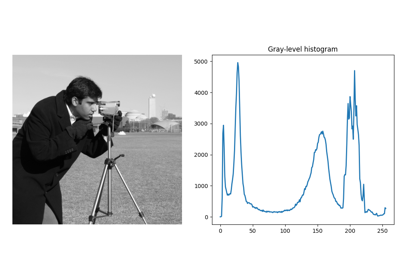Rank filters

## opening¶

skimage.morphology.opening(image, selem=None, out=None)[source]

Return greyscale morphological opening of an image.

The morphological opening on an image is defined as an erosion followed by a dilation. Opening can remove small bright spots (i.e. “salt”) and connect small dark cracks. This tends to “open” up (dark) gaps between (bright) features.

Parameters: image : ndarray Image array. selem : ndarray, optional The neighborhood expressed as an array of 1’s and 0’s. If None, use cross-shaped structuring element (connectivity=1). out : ndarray, optional The array to store the result of the morphology. If None is passed, a new array will be allocated. opening : array, same shape and type as image The result of the morphological opening.

Examples

>>> # Open up gap between two bright regions (but also shrink regions)
>>> import numpy as np
>>> from skimage.morphology import square
>>> bad_connection = np.array([[1, 0, 0, 0, 1],
...                            [1, 1, 0, 1, 1],
...                            [1, 1, 1, 1, 1],
...                            [1, 1, 0, 1, 1],
...                            [1, 0, 0, 0, 1]], dtype=np.uint8)
array([[0, 0, 0, 0, 0],
[1, 1, 0, 1, 1],
[1, 1, 0, 1, 1],
[1, 1, 0, 1, 1],
[0, 0, 0, 0, 0]], dtype=uint8)


### Examples using skimage.morphology.opening¶Morphological Filtering

## closing¶

skimage.morphology.closing(image, selem=None, out=None)[source]

Return greyscale morphological closing of an image.

The morphological closing on an image is defined as a dilation followed by an erosion. Closing can remove small dark spots (i.e. “pepper”) and connect small bright cracks. This tends to “close” up (dark) gaps between (bright) features.

Parameters: image : ndarray Image array. selem : ndarray, optional The neighborhood expressed as an array of 1’s and 0’s. If None, use cross-shaped structuring element (connectivity=1). out : ndarray, optional The array to store the result of the morphology. If None, is passed, a new array will be allocated. closing : array, same shape and type as image The result of the morphological closing.

Examples

>>> # Close a gap between two bright lines
>>> import numpy as np
>>> from skimage.morphology import square
>>> broken_line = np.array([[0, 0, 0, 0, 0],
...                         [0, 0, 0, 0, 0],
...                         [1, 1, 0, 1, 1],
...                         [0, 0, 0, 0, 0],
...                         [0, 0, 0, 0, 0]], dtype=np.uint8)
>>> closing(broken_line, square(3))
array([[0, 0, 0, 0, 0],
[0, 0, 0, 0, 0],
[1, 1, 1, 1, 1],
[0, 0, 0, 0, 0],
[0, 0, 0, 0, 0]], dtype=uint8)


### Examples using skimage.morphology.closing¶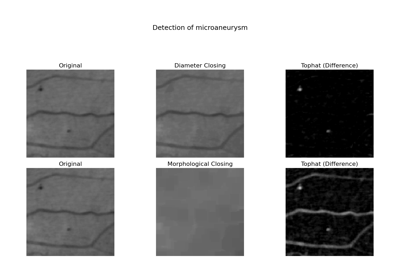Attribute operators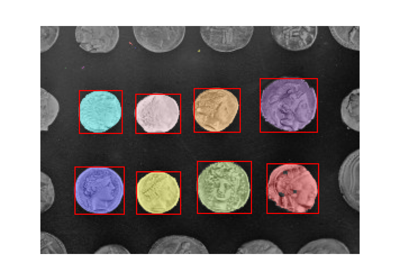Label image regionsMorphological Filtering

## white_tophat¶

skimage.morphology.white_tophat(image, selem=None, out=None)[source]

Return white top hat of an image.

The white top hat of an image is defined as the image minus its morphological opening. This operation returns the bright spots of the image that are smaller than the structuring element.

Parameters: image : ndarray Image array. selem : ndarray, optional The neighborhood expressed as an array of 1’s and 0’s. If None, use cross-shaped structuring element (connectivity=1). out : ndarray, optional The array to store the result of the morphology. If None is passed, a new array will be allocated. out : array, same shape and type as image The result of the morphological white top hat.

Examples

>>> # Subtract grey background from bright peak
>>> import numpy as np
>>> from skimage.morphology import square
>>> bright_on_grey = np.array([[2, 3, 3, 3, 2],
...                            [3, 4, 5, 4, 3],
...                            [3, 5, 9, 5, 3],
...                            [3, 4, 5, 4, 3],
...                            [2, 3, 3, 3, 2]], dtype=np.uint8)
>>> white_tophat(bright_on_grey, square(3))
array([[0, 0, 0, 0, 0],
[0, 0, 1, 0, 0],
[0, 1, 5, 1, 0],
[0, 0, 1, 0, 0],
[0, 0, 0, 0, 0]], dtype=uint8)


### Examples using skimage.morphology.white_tophat¶Morphological Filtering

## black_tophat¶

skimage.morphology.black_tophat(image, selem=None, out=None)[source]

Return black top hat of an image.

The black top hat of an image is defined as its morphological closing minus the original image. This operation returns the dark spots of the image that are smaller than the structuring element. Note that dark spots in the original image are bright spots after the black top hat.

Parameters: image : ndarray Image array. selem : ndarray, optional The neighborhood expressed as a 2-D array of 1’s and 0’s. If None, use cross-shaped structuring element (connectivity=1). out : ndarray, optional The array to store the result of the morphology. If None is passed, a new array will be allocated. out : array, same shape and type as image The result of the morphological black top hat.

Examples

>>> # Change dark peak to bright peak and subtract background
>>> import numpy as np
>>> from skimage.morphology import square
>>> dark_on_grey = np.array([[7, 6, 6, 6, 7],
...                          [6, 5, 4, 5, 6],
...                          [6, 4, 0, 4, 6],
...                          [6, 5, 4, 5, 6],
...                          [7, 6, 6, 6, 7]], dtype=np.uint8)
>>> black_tophat(dark_on_grey, square(3))
array([[0, 0, 0, 0, 0],
[0, 0, 1, 0, 0],
[0, 1, 5, 1, 0],
[0, 0, 1, 0, 0],
[0, 0, 0, 0, 0]], dtype=uint8)


### Examples using skimage.morphology.black_tophat¶Morphological Filtering

## square¶

skimage.morphology.square(width, dtype=<class 'numpy.uint8'>)[source]

Generates a flat, square-shaped structuring element.

Every pixel along the perimeter has a chessboard distance no greater than radius (radius=floor(width/2)) pixels.

Parameters: Returns: width : int The width and height of the square. selem : ndarray A structuring element consisting only of ones, i.e. every pixel belongs to the neighborhood. dtype : data-type The data type of the structuring element.

### Examples using skimage.morphology.square¶Attribute operatorsLabel image regions

## rectangle¶

skimage.morphology.rectangle(width, height, dtype=<class 'numpy.uint8'>)[source]

Generates a flat, rectangular-shaped structuring element.

Every pixel in the rectangle generated for a given width and given height belongs to the neighborhood.

Parameters: Returns: width : int The width of the rectangle. height : int The height of the rectangle. selem : ndarray A structuring element consisting only of ones, i.e. every pixel belongs to the neighborhood. dtype : data-type The data type of the structuring element.

## diamond¶

skimage.morphology.diamond(radius, dtype=<class 'numpy.uint8'>)[source]

Generates a flat, diamond-shaped structuring element.

A pixel is part of the neighborhood (i.e. labeled 1) if the city block/Manhattan distance between it and the center of the neighborhood is no greater than radius.

Parameters: Returns: radius : int The radius of the diamond-shaped structuring element. selem : ndarray The structuring element where elements of the neighborhood are 1 and 0 otherwise. dtype : data-type The data type of the structuring element.

## disk¶

skimage.morphology.disk(radius, dtype=<class 'numpy.uint8'>)[source]

Generates a flat, disk-shaped structuring element.

A pixel is within the neighborhood if the euclidean distance between it and the origin is no greater than radius.

Parameters: Returns: radius : int The radius of the disk-shaped structuring element. selem : ndarray The structuring element where elements of the neighborhood are 1 and 0 otherwise. dtype : data-type The data type of the structuring element.

### Examples using skimage.morphology.disk¶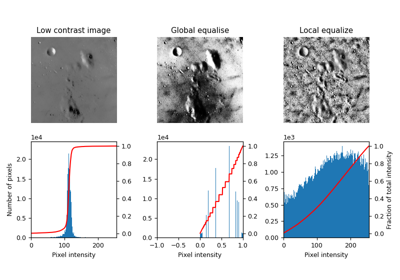Local Histogram Equalization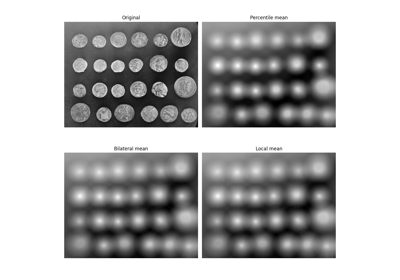Mean filters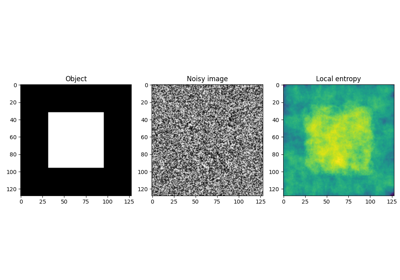Entropy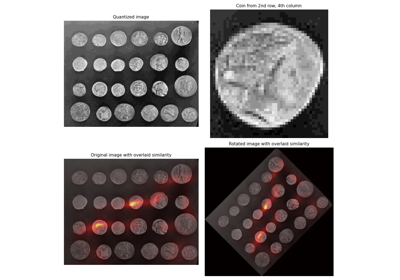Sliding window histogram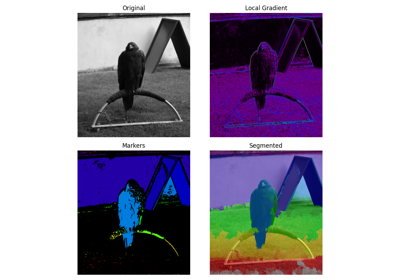Markers for watershed transformMorphological Filtering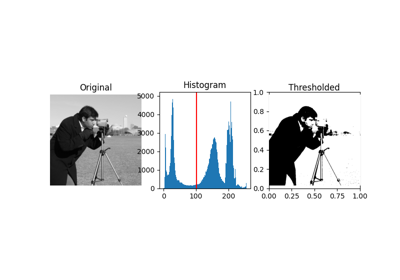ThresholdingRank filters

## cube¶

skimage.morphology.cube(width, dtype=<class 'numpy.uint8'>)[source]

Generates a cube-shaped structuring element.

This is the 3D equivalent of a square. Every pixel along the perimeter has a chessboard distance no greater than radius (radius=floor(width/2)) pixels.

Parameters: Returns: width : int The width, height and depth of the cube. selem : ndarray A structuring element consisting only of ones, i.e. every pixel belongs to the neighborhood. dtype : data-type The data type of the structuring element.

## octahedron¶

skimage.morphology.octahedron(radius, dtype=<class 'numpy.uint8'>)[source]

Generates a octahedron-shaped structuring element.

This is the 3D equivalent of a diamond. A pixel is part of the neighborhood (i.e. labeled 1) if the city block/Manhattan distance between it and the center of the neighborhood is no greater than radius.

Parameters: Returns: radius : int The radius of the octahedron-shaped structuring element. selem : ndarray The structuring element where elements of the neighborhood are 1 and 0 otherwise. dtype : data-type The data type of the structuring element.

## ball¶

skimage.morphology.ball(radius, dtype=<class 'numpy.uint8'>)[source]

Generates a ball-shaped structuring element.

This is the 3D equivalent of a disk. A pixel is within the neighborhood if the euclidean distance between it and the origin is no greater than radius.

Parameters: Returns: radius : int The radius of the ball-shaped structuring element. selem : ndarray The structuring element where elements of the neighborhood are 1 and 0 otherwise. dtype : data-type The data type of the structuring element.

## octagon¶

skimage.morphology.octagon(m, n, dtype=<class 'numpy.uint8'>)[source]

Generates an octagon shaped structuring element.

For a given size of (m) horizontal and vertical sides and a given (n) height or width of slanted sides octagon is generated. The slanted sides are 45 or 135 degrees to the horizontal axis and hence the widths and heights are equal.

Parameters: Returns: m : int The size of the horizontal and vertical sides. n : int The height or width of the slanted sides. selem : ndarray The structuring element where elements of the neighborhood are 1 and 0 otherwise. dtype : data-type The data type of the structuring element.

## star¶

skimage.morphology.star(a, dtype=<class 'numpy.uint8'>)[source]

Generates a star shaped structuring element.

Start has 8 vertices and is an overlap of square of size 2*a + 1 with its 45 degree rotated version. The slanted sides are 45 or 135 degrees to the horizontal axis.

Parameters: Returns: a : int Parameter deciding the size of the star structural element. The side of the square array returned is 2*a + 1 + 2*floor(a / 2). selem : ndarray The structuring element where elements of the neighborhood are 1 and 0 otherwise. dtype : data-type The data type of the structuring element.

## label¶

skimage.morphology.label(input, neighbors=None, background=None, return_num=False, connectivity=None)[source]

Label connected regions of an integer array.

Two pixels are connected when they are neighbors and have the same value. In 2D, they can be neighbors either in a 1- or 2-connected sense. The value refers to the maximum number of orthogonal hops to consider a pixel/voxel a neighbor:

1-connectivity      2-connectivity     diagonal connection close-up

[ ]           [ ]  [ ]  [ ]         [ ]
|               \  |  /             |  <- hop 2
[ ]--[x]--[ ]      [ ]--[x]--[ ]    [x]--[ ]
|               /  |  \         hop 1
[ ]           [ ]  [ ]  [ ]

Parameters: input : ndarray of dtype int Image to label. neighbors : {4, 8}, int, optional Whether to use 4- or 8-“connectivity”. In 3D, 4-“connectivity” means connected pixels have to share face, whereas with 8-“connectivity”, they have to share only edge or vertex. Deprecated, use connectivity instead. background : int, optional Consider all pixels with this value as background pixels, and label them as 0. By default, 0-valued pixels are considered as background pixels. return_num : bool, optional Whether to return the number of assigned labels. connectivity : int, optional Maximum number of orthogonal hops to consider a pixel/voxel as a neighbor. Accepted values are ranging from 1 to input.ndim. If None, a full connectivity of input.ndim is used. labels : ndarray of dtype int Labeled array, where all connected regions are assigned the same integer value. num : int, optional Number of labels, which equals the maximum label index and is only returned if return_num is True.

regionprops

References

  Christophe Fiorio and Jens Gustedt, “Two linear time Union-Find strategies for image processing”, Theoretical Computer Science 154 (1996), pp. 165-181.
  Kensheng Wu, Ekow Otoo and Arie Shoshani, “Optimizing connected component labeling algorithms”, Paper LBNL-56864, 2005, Lawrence Berkeley National Laboratory (University of California), http://repositories.cdlib.org/lbnl/LBNL-56864

Examples

>>> import numpy as np
>>> x = np.eye(3).astype(int)
>>> print(x)
[[1 0 0]
[0 1 0]
[0 0 1]]
>>> print(label(x, connectivity=1))
[[1 0 0]
[0 2 0]
[0 0 3]]
>>> print(label(x, connectivity=2))
[[1 0 0]
[0 1 0]
[0 0 1]]
>>> print(label(x, background=-1))
[[1 2 2]
[2 1 2]
[2 2 1]]
>>> x = np.array([[1, 0, 0],
...               [1, 1, 5],
...               [0, 0, 0]])
>>> print(label(x))
[[1 0 0]
[1 1 2]
[0 0 0]]


## watershed¶

skimage.morphology.watershed(image, markers=None, connectivity=1, offset=None, mask=None, compactness=0, watershed_line=False)[source]

Find watershed basins in image flooded from given markers.

Parameters: image: ndarray (2-D, 3-D, …) of integers Data array where the lowest value points are labeled first. markers: int, or ndarray of int, same shape as image, optional The desired number of markers, or an array marking the basins with the values to be assigned in the label matrix. Zero means not a marker. If None (no markers given), the local minima of the image are used as markers. connectivity: ndarray, optional An array with the same number of dimensions as image whose non-zero elements indicate neighbors for connection. Following the scipy convention, default is a one-connected array of the dimension of the image. offset: array_like of shape image.ndim, optional offset of the connectivity (one offset per dimension) mask: ndarray of bools or 0s and 1s, optional Array of same shape as image. Only points at which mask == True will be labeled. compactness : float, optional Use compact watershed  with given compactness parameter. Higher values result in more regularly-shaped watershed basins. watershed_line : bool, optional If watershed_line is True, a one-pixel wide line separates the regions obtained by the watershed algorithm. The line has the label 0. out: ndarray A labeled matrix of the same type and shape as markers

skimage.segmentation.random_walker
random walker segmentation A segmentation algorithm based on anisotropic diffusion, usually slower than the watershed but with good results on noisy data and boundaries with holes.

Notes

This function implements a watershed algorithm   that apportions pixels into marked basins. The algorithm uses a priority queue to hold the pixels with the metric for the priority queue being pixel value, then the time of entry into the queue - this settles ties in favor of the closest marker.

Some ideas taken from Soille, “Automated Basin Delineation from Digital Elevation Models Using Mathematical Morphology”, Signal Processing 20 (1990) 171-182

The most important insight in the paper is that entry time onto the queue solves two problems: a pixel should be assigned to the neighbor with the largest gradient or, if there is no gradient, pixels on a plateau should be split between markers on opposite sides.

This implementation converts all arguments to specific, lowest common denominator types, then passes these to a C algorithm.

Markers can be determined manually, or automatically using for example the local minima of the gradient of the image, or the local maxima of the distance function to the background for separating overlapping objects (see example).

References

  (1, 2) Peer Neubert & Peter Protzel (2014). Compact Watershed and Preemptive SLIC: On Improving Trade-offs of Superpixel Segmentation Algorithms. ICPR 2014, pp 996-1001. DOI:10.1109/ICPR.2014.181 https://www.tu-chemnitz.de/etit/proaut/forschung/rsrc/cws_pSLIC_ICPR.pdf

Examples

The watershed algorithm is useful to separate overlapping objects.

We first generate an initial image with two overlapping circles:

>>> x, y = np.indices((80, 80))
>>> x1, y1, x2, y2 = 28, 28, 44, 52
>>> r1, r2 = 16, 20
>>> mask_circle1 = (x - x1)**2 + (y - y1)**2 < r1**2
>>> mask_circle2 = (x - x2)**2 + (y - y2)**2 < r2**2


Next, we want to separate the two circles. We generate markers at the maxima of the distance to the background:

>>> from scipy import ndimage as ndi
>>> distance = ndi.distance_transform_edt(image)
>>> from skimage.feature import peak_local_max
>>> local_maxi = peak_local_max(distance, labels=image,
...                             footprint=np.ones((3, 3)),
...                             indices=False)
>>> markers = ndi.label(local_maxi)


Finally, we run the watershed on the image and markers:

>>> labels = watershed(-distance, markers, mask=image)


The algorithm works also for 3-D images, and can be used for example to separate overlapping spheres.

### Examples using skimage.morphology.watershed¶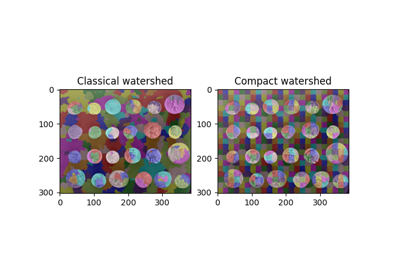Find Regular Segments Using Compact Watershed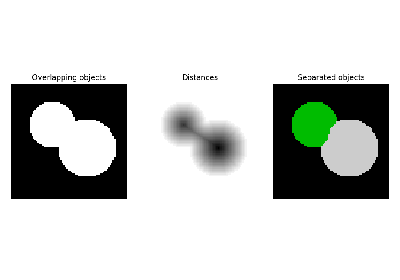Watershed segmentationMarkers for watershed transform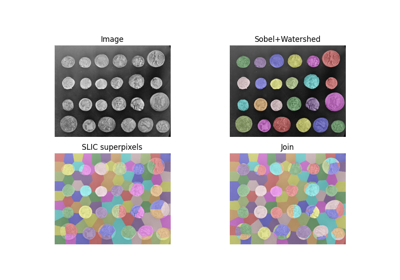Find the intersection of two segmentations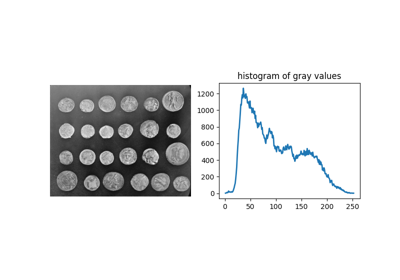Comparing edge-based and region-based segmentation

## skeletonize¶

skimage.morphology.skeletonize(image)[source]

Return the skeleton of a binary image.

Thinning is used to reduce each connected component in a binary image to a single-pixel wide skeleton.

Parameters: image : numpy.ndarray A binary image containing the objects to be skeletonized. ‘1’ represents foreground, and ‘0’ represents background. It also accepts arrays of boolean values where True is foreground. skeleton : ndarray A matrix containing the thinned image.

Notes

The algorithm [Zha84] works by making successive passes of the image, removing pixels on object borders. This continues until no more pixels can be removed. The image is correlated with a mask that assigns each pixel a number in the range [0…255] corresponding to each possible pattern of its 8 neighbouring pixels. A look up table is then used to assign the pixels a value of 0, 1, 2 or 3, which are selectively removed during the iterations.

Note that this algorithm will give different results than a medial axis transform, which is also often referred to as “skeletonization”.

References

 [Zha84] (1, 2) A fast parallel algorithm for thinning digital patterns, T. Y. Zhang and C. Y. Suen, Communications of the ACM, March 1984, Volume 27, Number 3.

Examples

>>> X, Y = np.ogrid[0:9, 0:9]
>>> ellipse = (1./3 * (X - 4)**2 + (Y - 4)**2 < 3**2).astype(np.uint8)
>>> ellipse
array([[0, 0, 0, 1, 1, 1, 0, 0, 0],
[0, 0, 1, 1, 1, 1, 1, 0, 0],
[0, 0, 1, 1, 1, 1, 1, 0, 0],
[0, 0, 1, 1, 1, 1, 1, 0, 0],
[0, 0, 1, 1, 1, 1, 1, 0, 0],
[0, 0, 1, 1, 1, 1, 1, 0, 0],
[0, 0, 1, 1, 1, 1, 1, 0, 0],
[0, 0, 1, 1, 1, 1, 1, 0, 0],
[0, 0, 0, 1, 1, 1, 0, 0, 0]], dtype=uint8)
>>> skel = skeletonize(ellipse)
>>> skel.astype(np.uint8)
array([[0, 0, 0, 0, 0, 0, 0, 0, 0],
[0, 0, 0, 0, 0, 0, 0, 0, 0],
[0, 0, 0, 0, 0, 0, 0, 0, 0],
[0, 0, 0, 0, 1, 0, 0, 0, 0],
[0, 0, 0, 0, 1, 0, 0, 0, 0],
[0, 0, 0, 0, 1, 0, 0, 0, 0],
[0, 0, 0, 0, 1, 0, 0, 0, 0],
[0, 0, 0, 0, 0, 0, 0, 0, 0],
[0, 0, 0, 0, 0, 0, 0, 0, 0]], dtype=uint8)


### Examples using skimage.morphology.skeletonize¶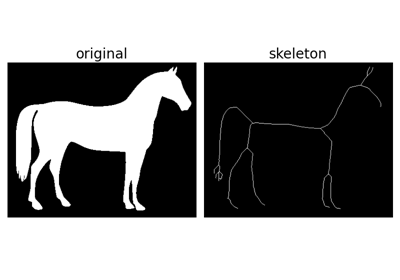SkeletonizeMorphological Filtering

## skeletonize_3d¶

skimage.morphology.skeletonize_3d(img)[source]

Compute the skeleton of a binary image.

Thinning is used to reduce each connected component in a binary image to a single-pixel wide skeleton.

Parameters: img : ndarray, 2D or 3D A binary image containing the objects to be skeletonized. Zeros represent background, nonzero values are foreground. skeleton : ndarray The thinned image.

Notes

The method of [Lee94] uses an octree data structure to examine a 3x3x3 neighborhood of a pixel. The algorithm proceeds by iteratively sweeping over the image, and removing pixels at each iteration until the image stops changing. Each iteration consists of two steps: first, a list of candidates for removal is assembled; then pixels from this list are rechecked sequentially, to better preserve connectivity of the image.

The algorithm this function implements is different from the algorithms used by either skeletonize or medial_axis, thus for 2D images the results produced by this function are generally different.

References

 [Lee94] (1, 2) T.-C. Lee, R.L. Kashyap and C.-N. Chu, Building skeleton models via 3-D medial surface/axis thinning algorithms. Computer Vision, Graphics, and Image Processing, 56(6):462-478, 1994.

### Examples using skimage.morphology.skeletonize_3d¶Skeletonize

## thin¶

skimage.morphology.thin(image, max_iter=None)[source]

Perform morphological thinning of a binary image.

Parameters: image : binary (M, N) ndarray The image to be thinned. max_iter : int, number of iterations, optional Regardless of the value of this parameter, the thinned image is returned immediately if an iteration produces no change. If this parameter is specified it thus sets an upper bound on the number of iterations performed. out : ndarray of bool Thinned image.

Notes

This algorithm  works by making multiple passes over the image, removing pixels matching a set of criteria designed to thin connected regions while preserving eight-connected components and 2 x 2 squares . In each of the two sub-iterations the algorithm correlates the intermediate skeleton image with a neighborhood mask, then looks up each neighborhood in a lookup table indicating whether the central pixel should be deleted in that sub-iteration.

References

  (1, 2) Z. Guo and R. W. Hall, “Parallel thinning with two-subiteration algorithms,” Comm. ACM, vol. 32, no. 3, pp. 359-373, 1989. DOI:10.1145/62065.62074
  (1, 2) Lam, L., Seong-Whan Lee, and Ching Y. Suen, “Thinning Methodologies-A Comprehensive Survey,” IEEE Transactions on Pattern Analysis and Machine Intelligence, Vol 14, No. 9, p. 879, 1992. DOI:10.1109/34.161346

Examples

>>> square = np.zeros((7, 7), dtype=np.uint8)
>>> square[1:-1, 2:-2] = 1
>>> square[0, 1] =  1
>>> square
array([[0, 1, 0, 0, 0, 0, 0],
[0, 0, 1, 1, 1, 0, 0],
[0, 0, 1, 1, 1, 0, 0],
[0, 0, 1, 1, 1, 0, 0],
[0, 0, 1, 1, 1, 0, 0],
[0, 0, 1, 1, 1, 0, 0],
[0, 0, 0, 0, 0, 0, 0]], dtype=uint8)
>>> skel = thin(square)
>>> skel.astype(np.uint8)
array([[0, 1, 0, 0, 0, 0, 0],
[0, 0, 1, 0, 0, 0, 0],
[0, 0, 0, 1, 0, 0, 0],
[0, 0, 0, 1, 0, 0, 0],
[0, 0, 0, 1, 0, 0, 0],
[0, 0, 0, 0, 0, 0, 0],
[0, 0, 0, 0, 0, 0, 0]], dtype=uint8)


### Examples using skimage.morphology.thin¶Skeletonize

## medial_axis¶

skimage.morphology.medial_axis(image, mask=None, return_distance=False)[source]

Compute the medial axis transform of a binary image

Parameters: image : binary ndarray, shape (M, N) The image of the shape to be skeletonized. mask : binary ndarray, shape (M, N), optional If a mask is given, only those elements in image with a true value in mask are used for computing the medial axis. return_distance : bool, optional If true, the distance transform is returned as well as the skeleton. out : ndarray of bools Medial axis transform of the image dist : ndarray of ints, optional Distance transform of the image (only returned if return_distance is True)

Notes

This algorithm computes the medial axis transform of an image as the ridges of its distance transform.

The different steps of the algorithm are as follows
• A lookup table is used, that assigns 0 or 1 to each configuration of the 3x3 binary square, whether the central pixel should be removed or kept. We want a point to be removed if it has more than one neighbor and if removing it does not change the number of connected components.
• The distance transform to the background is computed, as well as the cornerness of the pixel.
• The foreground (value of 1) points are ordered by the distance transform, then the cornerness.
• A cython function is called to reduce the image to its skeleton. It processes pixels in the order determined at the previous step, and removes or maintains a pixel according to the lookup table. Because of the ordering, it is possible to process all pixels in only one pass.

Examples

>>> square = np.zeros((7, 7), dtype=np.uint8)
>>> square[1:-1, 2:-2] = 1
>>> square
array([[0, 0, 0, 0, 0, 0, 0],
[0, 0, 1, 1, 1, 0, 0],
[0, 0, 1, 1, 1, 0, 0],
[0, 0, 1, 1, 1, 0, 0],
[0, 0, 1, 1, 1, 0, 0],
[0, 0, 1, 1, 1, 0, 0],
[0, 0, 0, 0, 0, 0, 0]], dtype=uint8)
>>> medial_axis(square).astype(np.uint8)
array([[0, 0, 0, 0, 0, 0, 0],
[0, 0, 1, 0, 1, 0, 0],
[0, 0, 0, 1, 0, 0, 0],
[0, 0, 0, 1, 0, 0, 0],
[0, 0, 0, 1, 0, 0, 0],
[0, 0, 1, 0, 1, 0, 0],
[0, 0, 0, 0, 0, 0, 0]], dtype=uint8)


### Examples using skimage.morphology.medial_axis¶Skeletonize

## convex_hull_image¶

skimage.morphology.convex_hull_image(image, offset_coordinates=True, tolerance=1e-10)[source]

Compute the convex hull image of a binary image.

The convex hull is the set of pixels included in the smallest convex polygon that surround all white pixels in the input image.

Parameters: image : array Binary input image. This array is cast to bool before processing. offset_coordinates : bool, optional If True, a pixel at coordinate, e.g., (4, 7) will be represented by coordinates (3.5, 7), (4.5, 7), (4, 6.5), and (4, 7.5). This adds some “extent” to a pixel when computing the hull. tolerance : float, optional Tolerance when determining whether a point is inside the hull. Due to numerical floating point errors, a tolerance of 0 can result in some points erroneously being classified as being outside the hull. hull : (M, N) array of bool Binary image with pixels in convex hull set to True.

References

### Examples using skimage.morphology.convex_hull_image¶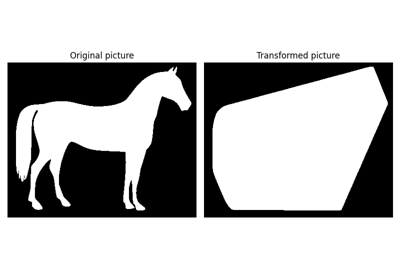Convex HullMorphological Filtering

## convex_hull_object¶

skimage.morphology.convex_hull_object(image, neighbors=8)[source]

Compute the convex hull image of individual objects in a binary image.

The convex hull is the set of pixels included in the smallest convex polygon that surround all white pixels in the input image.

Parameters: image : (M, N) array Binary input image. neighbors : {4, 8}, int Whether to use 4- or 8-connectivity. hull : ndarray of bool Binary image with pixels in convex hull set to True.

Notes

This function uses skimage.morphology.label to define unique objects, finds the convex hull of each using convex_hull_image, and combines these regions with logical OR. Be aware the convex hulls of unconnected objects may overlap in the result. If this is suspected, consider using convex_hull_image separately on each object.

## reconstruction¶

skimage.morphology.reconstruction(seed, mask, method='dilation', selem=None, offset=None)[source]

Perform a morphological reconstruction of an image.

Morphological reconstruction by dilation is similar to basic morphological dilation: high-intensity values will replace nearby low-intensity values. The basic dilation operator, however, uses a structuring element to determine how far a value in the input image can spread. In contrast, reconstruction uses two images: a “seed” image, which specifies the values that spread, and a “mask” image, which gives the maximum allowed value at each pixel. The mask image, like the structuring element, limits the spread of high-intensity values. Reconstruction by erosion is simply the inverse: low-intensity values spread from the seed image and are limited by the mask image, which represents the minimum allowed value.

Alternatively, you can think of reconstruction as a way to isolate the connected regions of an image. For dilation, reconstruction connects regions marked by local maxima in the seed image: neighboring pixels less-than-or-equal-to those seeds are connected to the seeded region. Local maxima with values larger than the seed image will get truncated to the seed value.

Parameters: seed : ndarray The seed image (a.k.a. marker image), which specifies the values that are dilated or eroded. mask : ndarray The maximum (dilation) / minimum (erosion) allowed value at each pixel. method : {‘dilation’|’erosion’}, optional Perform reconstruction by dilation or erosion. In dilation (or erosion), the seed image is dilated (or eroded) until limited by the mask image. For dilation, each seed value must be less than or equal to the corresponding mask value; for erosion, the reverse is true. Default is ‘dilation’. selem : ndarray, optional The neighborhood expressed as an n-D array of 1’s and 0’s. Default is the n-D square of radius equal to 1 (i.e. a 3x3 square for 2D images, a 3x3x3 cube for 3D images, etc.) offset : ndarray, optional The coordinates of the center of the structuring element. Default is located on the geometrical center of the selem, in that case selem dimensions must be odd. reconstructed : ndarray The result of morphological reconstruction.

Notes

The algorithm is taken from . Applications for greyscale reconstruction are discussed in  and .

References

  (1, 2) Robinson, “Efficient morphological reconstruction: a downhill filter”, Pattern Recognition Letters 25 (2004) 1759-1767.
  (1, 2) Vincent, L., “Morphological Grayscale Reconstruction in Image Analysis: Applications and Efficient Algorithms”, IEEE Transactions on Image Processing (1993)
  (1, 2) Soille, P., “Morphological Image Analysis: Principles and Applications”, Chapter 6, 2nd edition (2003), ISBN 3540429883.

Examples

>>> import numpy as np
>>> from skimage.morphology import reconstruction


First, we create a sinusoidal mask image with peaks at middle and ends.

>>> x = np.linspace(0, 4 * np.pi)


Then, we create a seed image initialized to the minimum mask value (for reconstruction by dilation, min-intensity values don’t spread) and add “seeds” to the left and right peak, but at a fraction of peak value (1).

>>> y_seed = y_mask.min() * np.ones_like(x)
>>> y_seed = 0.5
>>> y_seed[-1] = 0


The reconstructed image (or curve, in this case) is exactly the same as the mask image, except that the peaks are truncated to 0.5 and 0. The middle peak disappears completely: Since there were no seed values in this peak region, its reconstructed value is truncated to the surrounding value (-1).

As a more practical example, we try to extract the bright features of an image by subtracting a background image created by reconstruction.

>>> y, x = np.mgrid[:20:0.5, :20:0.5]
>>> bumps = np.sin(x) + np.sin(y)


To create the background image, set the mask image to the original image, and the seed image to the original image with an intensity offset, h.

>>> h = 0.3
>>> seed = bumps - h
>>> background = reconstruction(seed, bumps)


The resulting reconstructed image looks exactly like the original image, but with the peaks of the bumps cut off. Subtracting this reconstructed image from the original image leaves just the peaks of the bumps

>>> hdome = bumps - background


This operation is known as the h-dome of the image and leaves features of height h in the subtracted image.

### Examples using skimage.morphology.reconstruction¶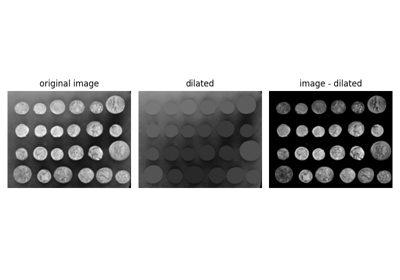Filtering regional maxima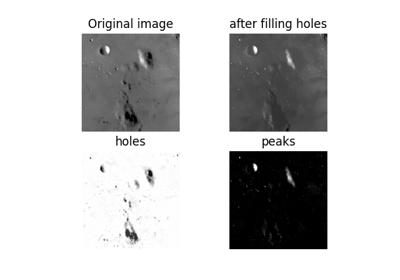Filling holes and finding peaks

## remove_small_objects¶

skimage.morphology.remove_small_objects(ar, min_size=64, connectivity=1, in_place=False)[source]

Remove objects smaller than the specified size.

Expects ar to be an array with labeled objects, and removes objects smaller than min_size. If ar is bool, the image is first labeled. This leads to potentially different behavior for bool and 0-and-1 arrays.

Parameters: ar : ndarray (arbitrary shape, int or bool type) The array containing the objects of interest. If the array type is int, the ints must be non-negative. min_size : int, optional (default: 64) The smallest allowable object size. connectivity : int, {1, 2, …, ar.ndim}, optional (default: 1) The connectivity defining the neighborhood of a pixel. Used during labelling if ar is bool. in_place : bool, optional (default: False) If True, remove the objects in the input array itself. Otherwise, make a copy. out : ndarray, same shape and type as input ar The input array with small connected components removed. TypeError If the input array is of an invalid type, such as float or string. ValueError If the input array contains negative values.

Examples

>>> from skimage import morphology
>>> a = np.array([[0, 0, 0, 1, 0],
...               [1, 1, 1, 0, 0],
...               [1, 1, 1, 0, 1]], bool)
>>> b = morphology.remove_small_objects(a, 6)
>>> b
array([[False, False, False, False, False],
[ True,  True,  True, False, False],
[ True,  True,  True, False, False]], dtype=bool)
>>> c = morphology.remove_small_objects(a, 7, connectivity=2)
>>> c
array([[False, False, False,  True, False],
[ True,  True,  True, False, False],
[ True,  True,  True, False, False]], dtype=bool)
>>> d = morphology.remove_small_objects(a, 6, in_place=True)
>>> d is a
True


### Examples using skimage.morphology.remove_small_objects¶Comparing edge-based and region-based segmentation

## remove_small_holes¶

skimage.morphology.remove_small_holes(ar, area_threshold=64, connectivity=1, in_place=False, min_size=None)[source]

Remove contiguous holes smaller than the specified size.

Parameters: ar : ndarray (arbitrary shape, int or bool type) The array containing the connected components of interest. area_threshold : int, optional (default: 64) The maximum area, in pixels, of a contiguous hole that will be filled. Replaces min_size. connectivity : int, {1, 2, …, ar.ndim}, optional (default: 1) The connectivity defining the neighborhood of a pixel. in_place : bool, optional (default: False) If True, remove the connected components in the input array itself. Otherwise, make a copy. out : ndarray, same shape and type as input ar The input array with small holes within connected components removed. TypeError If the input array is of an invalid type, such as float or string. ValueError If the input array contains negative values.

Notes

If the array type is int, it is assumed that it contains already-labeled objects. The labels are not kept in the output image (this function always outputs a bool image). It is suggested that labeling is completed after using this function.

Examples

>>> from skimage import morphology
>>> a = np.array([[1, 1, 1, 1, 1, 0],
...               [1, 1, 1, 0, 1, 0],
...               [1, 0, 0, 1, 1, 0],
...               [1, 1, 1, 1, 1, 0]], bool)
>>> b = morphology.remove_small_holes(a, 2)
>>> b
array([[ True,  True,  True,  True,  True, False],
[ True,  True,  True,  True,  True, False],
[ True, False, False,  True,  True, False],
[ True,  True,  True,  True,  True, False]], dtype=bool)
>>> c = morphology.remove_small_holes(a, 2, connectivity=2)
>>> c
array([[ True,  True,  True,  True,  True, False],
[ True,  True,  True, False,  True, False],
[ True, False, False,  True,  True, False],
[ True,  True,  True,  True,  True, False]], dtype=bool)
>>> d = morphology.remove_small_holes(a, 2, in_place=True)
>>> d is a
True


## h_minima¶

skimage.morphology.h_minima(image, h, selem=None)[source]

Determine all minima of the image with depth >= h.

The local minima are defined as connected sets of pixels with equal grey level strictly smaller than the grey levels of all pixels in direct neighborhood of the set.

A local minimum M of depth h is a local minimum for which there is at least one path joining M with a deeper minimum on which the maximal value is f(M) + h (i.e. the values along the path are not increasing by more than h with respect to the minimum’s value) and no path for which the maximal value is smaller.

Parameters: image : ndarray The input image for which the minima are to be calculated. h : unsigned integer The minimal depth of all extracted minima. selem : ndarray, optional The neighborhood expressed as an n-D array of 1’s and 0’s. Default is the ball of radius 1 according to the maximum norm (i.e. a 3x3 square for 2D images, a 3x3x3 cube for 3D images, etc.) h_min : ndarray The minima of depth >= h. The result image is a binary image, where pixels belonging to the selected minima take value 1, the other pixels take value 0.

skimage.morphology.extrema.h_maxima, skimage.morphology.extrema.local_maxima, skimage.morphology.extrema.local_minima

References

  Soille, P., “Morphological Image Analysis: Principles and Applications” (Chapter 6), 2nd edition (2003), ISBN 3540429883.

Examples

>>> import numpy as np
>>> from skimage.morphology import extrema


We create an image (quadratic function with a minimum in the center and 4 additional constant maxima. The depth of the minima are: 1, 21, 41, 61, 81, 101

>>> w = 10
>>> x, y = np.mgrid[0:w,0:w]
>>> f = 180 + 0.2*((x - w/2)**2 + (y-w/2)**2)
>>> f[2:4,2:4] = 160; f[2:4,7:9] = 140; f[7:9,2:4] = 120; f[7:9,7:9] = 100
>>> f = f.astype(np.int)


We can calculate all minima with a depth of at least 40:

>>> minima = extrema.h_minima(f, 40)


The resulting image will contain 4 local minima.

## h_maxima¶

skimage.morphology.h_maxima(image, h, selem=None)[source]

Determine all maxima of the image with height >= h.

The local maxima are defined as connected sets of pixels with equal grey level strictly greater than the grey level of all pixels in direct neighborhood of the set.

A local maximum M of height h is a local maximum for which there is at least one path joining M with a higher maximum on which the minimal value is f(M) - h (i.e. the values along the path are not decreasing by more than h with respect to the maximum’s value) and no path for which the minimal value is greater.

Parameters: image : ndarray The input image for which the maxima are to be calculated. h : unsigned integer The minimal height of all extracted maxima. selem : ndarray, optional The neighborhood expressed as an n-D array of 1’s and 0’s. Default is the ball of radius 1 according to the maximum norm (i.e. a 3x3 square for 2D images, a 3x3x3 cube for 3D images, etc.) h_max : ndarray The maxima of height >= h. The result image is a binary image, where pixels belonging to the selected maxima take value 1, the others take value 0.

skimage.morphology.extrema.h_minima, skimage.morphology.extrema.local_maxima, skimage.morphology.extrema.local_minima

References

  Soille, P., “Morphological Image Analysis: Principles and Applications” (Chapter 6), 2nd edition (2003), ISBN 3540429883.

Examples

>>> import numpy as np
>>> from skimage.morphology import extrema


We create an image (quadratic function with a maximum in the center and 4 additional constant maxima. The heights of the maxima are: 1, 21, 41, 61, 81, 101

>>> w = 10
>>> x, y = np.mgrid[0:w,0:w]
>>> f = 20 - 0.2*((x - w/2)**2 + (y-w/2)**2)
>>> f[2:4,2:4] = 40; f[2:4,7:9] = 60; f[7:9,2:4] = 80; f[7:9,7:9] = 100
>>> f = f.astype(np.int)


We can calculate all maxima with a height of at least 40:

>>> maxima = extrema.h_maxima(f, 40)


The resulting image will contain 4 local maxima.

### Examples using skimage.morphology.h_maxima¶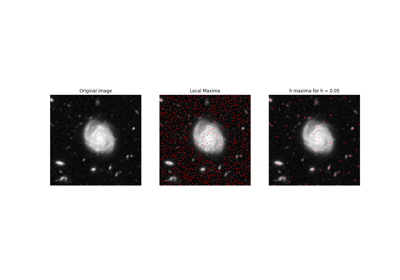Extrema

## local_maxima¶

skimage.morphology.local_maxima(image, selem=None, connectivity=None, indices=False, allow_borders=True)[source]

Find local maxima of n-dimensional array.

The local maxima are defined as connected sets of pixels with equal gray level (plateaus) strictly greater than the gray levels of all pixels in the neighborhood.

Parameters: image : ndarray An n-dimensional array. selem : ndarray, optional A structuring element used to determine the neighborhood of each evaluated pixel (True denotes a connected pixel). It must be a boolean array and have the same number of dimensions as image. If neither selem nor connectivity are given, all adjacent pixels are considered as part of the neighborhood. connectivity : int, optional A number used to determine the neighborhood of each evaluated pixel. Adjacent pixels whose squared distance from the center is less than or equal to connectivity are considered neighbors. Ignored if selem is not None. indices : bool, optional If True, the output will be a tuple of one-dimensional arrays representing the indices of local maxima in each dimension. If False, the output will be a boolean array with the same shape as image. allow_borders : bool, optional If true, plateaus that touch the image border are valid maxima. maxima : ndarray or tuple[ndarray] If indices is false, a boolean array with the same shape as image is returned with True indicating the position of local maxima (False otherwise). If indices is true, a tuple of one-dimensional arrays containing the coordinates (indices) of all found maxima. UserWarning If allow_borders is false and any dimension of the given image is shorter than 3 samples, maxima can’t exist and a warning is shown.

Notes

This function operates on the following ideas:

1. Make a first pass over the image’s last dimension and flag candidates for local maxima by comparing pixels in only one direction. If the pixels aren’t connected in the last dimension all pixels are flagged as candidates instead.

For each candidate:

1. Perform a flood-fill to find all connected pixels that have the same gray value and are part of the plateau.
2. Consider the connected neighborhood of a plateau: if no bordering sample has a higher gray level, mark the plateau as a definite local maximum.

Examples

>>> from skimage.morphology import local_maxima
>>> image = np.zeros((4, 7), dtype=int)
>>> image[1:3, 1:3] = 1
>>> image[3, 0] = 1
>>> image[1:3, 4:6] = 2
>>> image[3, 6] = 3
>>> image
array([[0, 0, 0, 0, 0, 0, 0],
[0, 1, 1, 0, 2, 2, 0],
[0, 1, 1, 0, 2, 2, 0],
[1, 0, 0, 0, 0, 0, 3]])


Find local maxima by comparing to all neighboring pixels (maximal connectivity):

>>> local_maxima(image)
array([[False, False, False, False, False, False, False],
[False,  True,  True, False, False, False, False],
[False,  True,  True, False, False, False, False],
[ True, False, False, False, False, False,  True]], dtype=bool)
>>> local_maxima(image, indices=True)
(array([1, 1, 2, 2, 3, 3]), array([1, 2, 1, 2, 0, 6]))


Find local maxima without comparing to diagonal pixels (connectivity 1):

>>> local_maxima(image, connectivity=1)
array([[False, False, False, False, False, False, False],
[False,  True,  True, False,  True,  True, False],
[False,  True,  True, False,  True,  True, False],
[ True, False, False, False, False, False,  True]], dtype=bool)


and exclude maxima that border the image edge:

>>> local_maxima(image, connectivity=1, allow_borders=False)
array([[False, False, False, False, False, False, False],
[False,  True,  True, False,  True,  True, False],
[False,  True,  True, False,  True,  True, False],
[False, False, False, False, False, False, False]], dtype=bool)


### Examples using skimage.morphology.local_maxima¶Extrema

## local_minima¶

skimage.morphology.local_minima(image, selem=None, connectivity=None, indices=False, allow_borders=True)[source]

Find local minima of n-dimensional array.

The local minima are defined as connected sets of pixels with equal gray level (plateaus) strictly smaller than the gray levels of all pixels in the neighborhood.

Parameters: image : ndarray An n-dimensional array. selem : ndarray, optional A structuring element used to determine the neighborhood of each evaluated pixel (True denotes a connected pixel). It must be a boolean array and have the same number of dimensions as image. If neither selem nor connectivity are given, all adjacent pixels are considered as part of the neighborhood. connectivity : int, optional A number used to determine the neighborhood of each evaluated pixel. Adjacent pixels whose squared distance from the center is less than or equal to connectivity are considered neighbors. Ignored if selem is not None. indices : bool, optional If True, the output will be a tuple of one-dimensional arrays representing the indices of local minima in each dimension. If False, the output will be a boolean array with the same shape as image. allow_borders : bool, optional If true, plateaus that touch the image border are valid minima. minima : ndarray or tuple[ndarray] If indices is false, a boolean array with the same shape as image is returned with True indicating the position of local minima (False otherwise). If indices is true, a tuple of one-dimensional arrays containing the coordinates (indices) of all found minima.

Notes

This function operates on the following ideas:

1. Make a first pass over the image’s last dimension and flag candidates for local minima by comparing pixels in only one direction. If the pixels aren’t connected in the last dimension all pixels are flagged as candidates instead.

For each candidate:

1. Perform a flood-fill to find all connected pixels that have the same gray value and are part of the plateau.
2. Consider the connected neighborhood of a plateau: if no bordering sample has a smaller gray level, mark the plateau as a definite local minimum.

Examples

>>> from skimage.morphology import local_minima
>>> image = np.zeros((4, 7), dtype=int)
>>> image[1:3, 1:3] = -1
>>> image[3, 0] = -1
>>> image[1:3, 4:6] = -2
>>> image[3, 6] = -3
>>> image
array([[ 0,  0,  0,  0,  0,  0,  0],
[ 0, -1, -1,  0, -2, -2,  0],
[ 0, -1, -1,  0, -2, -2,  0],
[-1,  0,  0,  0,  0,  0, -3]])


Find local minima by comparing to all neighboring pixels (maximal connectivity):

>>> local_minima(image)
array([[False, False, False, False, False, False, False],
[False,  True,  True, False, False, False, False],
[False,  True,  True, False, False, False, False],
[ True, False, False, False, False, False,  True]], dtype=bool)
>>> local_minima(image, indices=True)
(array([1, 1, 2, 2, 3, 3]), array([1, 2, 1, 2, 0, 6]))


Find local minima without comparing to diagonal pixels (connectivity 1):

>>> local_minima(image, connectivity=1)
array([[False, False, False, False, False, False, False],
[False,  True,  True, False,  True,  True, False],
[False,  True,  True, False,  True,  True, False],
[ True, False, False, False, False, False,  True]], dtype=bool)


and exclude minima that border the image edge:

>>> local_minima(image, connectivity=1, allow_borders=False)
array([[False, False, False, False, False, False, False],
[False,  True,  True, False,  True,  True, False],
[False,  True,  True, False,  True,  True, False],
[False, False, False, False, False, False, False]], dtype=bool)


## flood¶

skimage.morphology.flood(image, seed_point, *, selem=None, connectivity=None, tolerance=None)[source]

Mask corresponding to a flood fill.

Starting at a specific seed_point, connected points equal or within tolerance of the seed value are found.

Parameters: image : ndarray An n-dimensional array. seed_point : tuple or int The point in image used as the starting point for the flood fill. If the image is 1D, this point may be given as an integer. selem : ndarray, optional A structuring element used to determine the neighborhood of each evaluated pixel. It must contain only 1’s and 0’s, have the same number of dimensions as image. If not given, all adjacent pixels are considered as part of the neighborhood (fully connected). connectivity : int, optional A number used to determine the neighborhood of each evaluated pixel. Adjacent pixels whose squared distance from the center is larger or equal to connectivity are considered neighbors. Ignored if selem is not None. tolerance : float or int, optional If None (default), adjacent values must be strictly equal to the initial value of image at seed_point. This is fastest. If a value is given, a comparison will be done at every point and if within tolerance of the initial value will also be filled (inclusive). mask : ndarray A Boolean array with the same shape as image is returned, with True values for areas connected to and equal (or within tolerance of) the seed point. All other values are False.

Notes

The conceptual analogy of this operation is the ‘paint bucket’ tool in many raster graphics programs. This function returns just the mask representing the fill.

If indices are desired rather than masks for memory reasons, the user can simply run numpy.nonzero on the result, save the indices, and discard this mask.

Examples

>>> from skimage.morphology import flood
>>> image = np.zeros((4, 7), dtype=int)
>>> image[1:3, 1:3] = 1
>>> image[3, 0] = 1
>>> image[1:3, 4:6] = 2
>>> image[3, 6] = 3
>>> image
array([[0, 0, 0, 0, 0, 0, 0],
[0, 1, 1, 0, 2, 2, 0],
[0, 1, 1, 0, 2, 2, 0],
[1, 0, 0, 0, 0, 0, 3]])


Fill connected ones with 5, with full connectivity (diagonals included):

>>> mask = flood(image, (1, 1))
>>> image_flooded = image.copy()
>>> image_flooded
array([[0, 0, 0, 0, 0, 0, 0],
[0, 5, 5, 0, 2, 2, 0],
[0, 5, 5, 0, 2, 2, 0],
[5, 0, 0, 0, 0, 0, 3]])


Fill connected ones with 5, excluding diagonal points (connectivity 1):

>>> mask = flood(image, (1, 1), connectivity=1)
>>> image_flooded = image.copy()
>>> image_flooded
array([[0, 0, 0, 0, 0, 0, 0],
[0, 5, 5, 0, 2, 2, 0],
[0, 5, 5, 0, 2, 2, 0],
[1, 0, 0, 0, 0, 0, 3]])


Fill with a tolerance:

>>> mask = flood(image, (0, 0), tolerance=1)
>>> image_flooded = image.copy()
>>> image_flooded
array([[5, 5, 5, 5, 5, 5, 5],
[5, 5, 5, 5, 2, 2, 5],
[5, 5, 5, 5, 2, 2, 5],
[5, 5, 5, 5, 5, 5, 3]])


## flood_fill¶

skimage.morphology.flood_fill(image, seed_point, new_value, *, selem=None, connectivity=None, tolerance=None, inplace=False)[source]

Perform flood filling on an image.

Starting at a specific seed_point, connected points equal or within tolerance of the seed value are found, then set to new_value.

Parameters: image : ndarray An n-dimensional array. seed_point : tuple or int The point in image used as the starting point for the flood fill. If the image is 1D, this point may be given as an integer. new_value : image type New value to set the entire fill. This must be chosen in agreement with the dtype of image. selem : ndarray, optional A structuring element used to determine the neighborhood of each evaluated pixel. It must contain only 1’s and 0’s, have the same number of dimensions as image. If not given, all adjacent pixels are considered as part of the neighborhood (fully connected). connectivity : int, optional A number used to determine the neighborhood of each evaluated pixel. Adjacent pixels whose squared distance from the center is less than or equal to connectivity are considered neighbors. Ignored if selem is not None. tolerance : float or int, optional If None (default), adjacent values must be strictly equal to the value of image at seed_point to be filled. This is fastest. If a tolerance is provided, adjacent points with values within plus or minus tolerance from the seed point are filled (inclusive). inplace : bool, optional If True, flood filling is applied to image inplace. If False, the flood filled result is returned without modifying the input image (default). filled : ndarray An array with the same shape as image is returned, with values in areas connected to and equal (or within tolerance of) the seed point replaced with new_value.

Notes

The conceptual analogy of this operation is the ‘paint bucket’ tool in many raster graphics programs.

Examples

>>> from skimage.morphology import flood_fill
>>> image = np.zeros((4, 7), dtype=int)
>>> image[1:3, 1:3] = 1
>>> image[3, 0] = 1
>>> image[1:3, 4:6] = 2
>>> image[3, 6] = 3
>>> image
array([[0, 0, 0, 0, 0, 0, 0],
[0, 1, 1, 0, 2, 2, 0],
[0, 1, 1, 0, 2, 2, 0],
[1, 0, 0, 0, 0, 0, 3]])


Fill connected ones with 5, with full connectivity (diagonals included):

>>> flood_fill(image, (1, 1), 5)
array([[0, 0, 0, 0, 0, 0, 0],
[0, 5, 5, 0, 2, 2, 0],
[0, 5, 5, 0, 2, 2, 0],
[5, 0, 0, 0, 0, 0, 3]])


Fill connected ones with 5, excluding diagonal points (connectivity 1):

>>> flood_fill(image, (1, 1), 5, connectivity=1)
array([[0, 0, 0, 0, 0, 0, 0],
[0, 5, 5, 0, 2, 2, 0],
[0, 5, 5, 0, 2, 2, 0],
[1, 0, 0, 0, 0, 0, 3]])


Fill with a tolerance:

>>> flood_fill(image, (0, 0), 5, tolerance=1)
array([[5, 5, 5, 5, 5, 5, 5],
[5, 5, 5, 5, 2, 2, 5],
[5, 5, 5, 5, 2, 2, 5],
[5, 5, 5, 5, 5, 5, 3]])


## max_tree¶

skimage.morphology.max_tree(image, connectivity=1)[source]

Build the max tree from an image.

Component trees represent the hierarchical structure of the connected components resulting from sequential thresholding operations applied to an image. A connected component at one level is parent of a component at a higher level if the latter is included in the first. A max-tree is an efficient representation of a component tree. A connected component at one level is represented by one reference pixel at this level, which is parent to all other pixels at that level and to the reference pixel at the level above. The max-tree is the basis for many morphological operators, namely connected operators.

Parameters: image: ndarray The input image for which the max-tree is to be calculated. This image can be of any type. connectivity: unsigned int, optional The neighborhood connectivity. The integer represents the maximum number of orthogonal steps to reach a neighbor. In 2D, it is 1 for a 4-neighborhood and 2 for a 8-neighborhood. Default value is 1. parent: ndarray, int64 Array of same shape as image. The value of each pixel is the index of its parent in the ravelled array. tree_traverser: 1D array, int64 The ordered pixel indices (referring to the ravelled array). The pixels are ordered such that every pixel is preceded by its parent (except for the root which has no parent).

References

  Salembier, P., Oliveras, A., & Garrido, L. (1998). Antiextensive Connected Operators for Image and Sequence Processing. IEEE Transactions on Image Processing, 7(4), 555-570. DOI:10.1109/83.663500
  Berger, C., Geraud, T., Levillain, R., Widynski, N., Baillard, A., Bertin, E. (2007). Effective Component Tree Computation with Application to Pattern Recognition in Astronomical Imaging. In International Conference on Image Processing (ICIP) (pp. 41-44). DOI:10.1109/ICIP.2007.4379949
  Najman, L., & Couprie, M. (2006). Building the component tree in quasi-linear time. IEEE Transactions on Image Processing, 15(11), 3531-3539. DOI:10.1109/TIP.2006.877518
  Carlinet, E., & Geraud, T. (2014). A Comparative Review of Component Tree Computation Algorithms. IEEE Transactions on Image Processing, 23(9), 3885-3895. DOI:10.1109/TIP.2014.2336551

Examples

We create a small sample image (Figure 1 from ) and build the max-tree.

>>> image = np.array([[15, 13, 16], [12, 12, 10], [16, 12, 14]])
>>> P, S = max_tree(image, connectivity=2)


### Examples using skimage.morphology.max_tree¶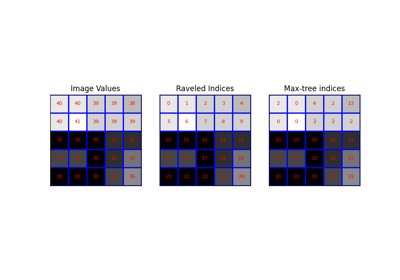Max-tree

## area_opening¶

skimage.morphology.area_opening(image, area_threshold=64, connectivity=1, parent=None, tree_traverser=None)[source]

Perform an area opening of the image.

Area opening removes all bright structures of an image with a surface smaller than area_threshold. The output image is thus the largest image smaller than the input for which all local maxima have at least a surface of area_threshold pixels.

Area openings are similar to morphological openings, but they do not use a fixed structuring element, but rather a deformable one, with surface = area_threshold. Consequently, the area_opening with area_threshold=1 is the identity.

In the binary case, area openings are equivalent to remove_small_objects; this operator is thus extended to gray-level images.

Technically, this operator is based on the max-tree representation of the image.

Parameters: image: ndarray The input image for which the area_opening is to be calculated. This image can be of any type. area_threshold: unsigned int The size parameter (number of pixels). The default value is arbitrarily chosen to be 64. connectivity: unsigned int, optional The neighborhood connectivity. The integer represents the maximum number of orthogonal steps to reach a neighbor. In 2D, it is 1 for a 4-neighborhood and 2 for a 8-neighborhood. Default value is 1. parent: ndarray, int64, optional Parent image representing the max tree of the image. The value of each pixel is the index of its parent in the ravelled array. tree_traverser: 1D array, int64, optional The ordered pixel indices (referring to the ravelled array). The pixels are ordered such that every pixel is preceded by its parent (except for the root which has no parent). output: ndarray Output image of the same shape and type as the input image.

References

  Vincent L., Proc. “Grayscale area openings and closings, their efficient implementation and applications”, EURASIP Workshop on Mathematical Morphology and its Applications to Signal Processing, Barcelona, Spain, pp.22-27, May 1993.
  Soille, P., “Morphological Image Analysis: Principles and Applications” (Chapter 6), 2nd edition (2003), ISBN 3540429883. DOI:10.1007/978-3-662-05088-0
  Salembier, P., Oliveras, A., & Garrido, L. (1998). Antiextensive Connected Operators for Image and Sequence Processing. IEEE Transactions on Image Processing, 7(4), 555-570. DOI:10.1109/83.663500
  Najman, L., & Couprie, M. (2006). Building the component tree in quasi-linear time. IEEE Transactions on Image Processing, 15(11), 3531-3539. DOI:10.1109/TIP.2006.877518
  Carlinet, E., & Geraud, T. (2014). A Comparative Review of Component Tree Computation Algorithms. IEEE Transactions on Image Processing, 23(9), 3885-3895. DOI:10.1109/TIP.2014.2336551

Examples

We create an image (quadratic function with a maximum in the center and 4 additional local maxima.

>>> w = 12
>>> x, y = np.mgrid[0:w,0:w]
>>> f = 20 - 0.2*((x - w/2)**2 + (y-w/2)**2)
>>> f[2:3,1:5] = 40; f[2:4,9:11] = 60; f[9:11,2:4] = 80
>>> f[9:10,9:11] = 100; f[10,10] = 100
>>> f = f.astype(np.int)


We can calculate the area opening:

>>> open = area_opening(f, 8, connectivity=1)


The peaks with a surface smaller than 8 are removed.

## area_closing¶

skimage.morphology.area_closing(image, area_threshold=64, connectivity=1, parent=None, tree_traverser=None)[source]

Perform an area closing of the image.

Area closing removes all dark structures of an image with a surface smaller than area_threshold. The output image is larger than or equal to the input image for every pixel and all local minima have at least a surface of area_threshold pixels.

Area closings are similar to morphological closings, but they do not use a fixed structuring element, but rather a deformable one, with surface = area_threshold.

In the binary case, area closings are equivalent to remove_small_holes; this operator is thus extended to gray-level images.

Technically, this operator is based on the max-tree representation of the image.

Parameters: image: ndarray The input image for which the area_closing is to be calculated. This image can be of any type. area_threshold: unsigned int The size parameter (number of pixels). The default value is arbitrarily chosen to be 64. connectivity: unsigned int, optional The neighborhood connectivity. The integer represents the maximum number of orthogonal steps to reach a neighbor. In 2D, it is 1 for a 4-neighborhood and 2 for a 8-neighborhood. Default value is 1. parent: ndarray, int64, optional Parent image representing the max tree of the inverted image. The value of each pixel is the index of its parent in the ravelled array. See Note for further details. tree_traverser: 1D array, int64, optional The ordered pixel indices (referring to the ravelled array). The pixels are ordered such that every pixel is preceded by its parent (except for the root which has no parent). output: ndarray Output image of the same shape and type as input image.

References

  Vincent L., Proc. “Grayscale area openings and closings, their efficient implementation and applications”, EURASIP Workshop on Mathematical Morphology and its Applications to Signal Processing, Barcelona, Spain, pp.22-27, May 1993.
  Soille, P., “Morphological Image Analysis: Principles and Applications” (Chapter 6), 2nd edition (2003), ISBN 3540429883. DOI:10.1007/978-3-662-05088-0
  Salembier, P., Oliveras, A., & Garrido, L. (1998). Antiextensive Connected Operators for Image and Sequence Processing. IEEE Transactions on Image Processing, 7(4), 555-570. DOI:10.1109/83.663500
  Najman, L., & Couprie, M. (2006). Building the component tree in quasi-linear time. IEEE Transactions on Image Processing, 15(11), 3531-3539. DOI:10.1109/TIP.2006.877518
  Carlinet, E., & Geraud, T. (2014). A Comparative Review of Component Tree Computation Algorithms. IEEE Transactions on Image Processing, 23(9), 3885-3895. DOI:10.1109/TIP.2014.2336551

Examples

We create an image (quadratic function with a minimum in the center and 4 additional local minima.

>>> w = 12
>>> x, y = np.mgrid[0:w,0:w]
>>> f = 180 + 0.2*((x - w/2)**2 + (y-w/2)**2)
>>> f[2:3,1:5] = 160; f[2:4,9:11] = 140; f[9:11,2:4] = 120
>>> f[9:10,9:11] = 100; f[10,10] = 100
>>> f = f.astype(np.int)


We can calculate the area closing:

>>> closed = area_closing(f, 8, connectivity=1)


All small minima are removed, and the remaining minima have at least a size of 8.

## diameter_opening¶

skimage.morphology.diameter_opening(image, diameter_threshold=8, connectivity=1, parent=None, tree_traverser=None)[source]

Perform a diameter opening of the image.

Diameter opening removes all bright structures of an image with maximal extension smaller than diameter_threshold. The maximal extension is defined as the maximal extension of the bounding box. The operator is also called Bounding Box Opening. In practice, the result is similar to a morphological opening, but long and thin structures are not removed.

Technically, this operator is based on the max-tree representation of the image.

Parameters: image: ndarray The input image for which the area_opening is to be calculated. This image can be of any type. diameter_threshold: unsigned int The maximal extension parameter (number of pixels). The default value is 8. connectivity: unsigned int, optional The neighborhood connectivity. The integer represents the maximum number of orthogonal steps to reach a neighbor. In 2D, it is 1 for a 4-neighborhood and 2 for a 8-neighborhood. Default value is 1. parent: ndarray, int64, optional Parent image representing the max tree of the image. The value of each pixel is the index of its parent in the ravelled array. tree_traverser: 1D array, int64, optional The ordered pixel indices (referring to the ravelled array). The pixels are ordered such that every pixel is preceded by its parent (except for the root which has no parent). output: ndarray Output image of the same shape and type as the input image.

References

  Walter, T., & Klein, J.-C. (2002). Automatic Detection of Microaneurysms in Color Fundus Images of the Human Retina by Means of the Bounding Box Closing. In A. Colosimo, P. Sirabella, A. Giuliani (Eds.), Medical Data Analysis. Lecture Notes in Computer Science, vol 2526, pp. 210-220. Springer Berlin Heidelberg. DOI:10.1007/3-540-36104-9_23
  Carlinet, E., & Geraud, T. (2014). A Comparative Review of Component Tree Computation Algorithms. IEEE Transactions on Image Processing, 23(9), 3885-3895. DOI:10.1109/TIP.2014.2336551

Examples

We create an image (quadratic function with a maximum in the center and 4 additional local maxima.

>>> w = 12
>>> x, y = np.mgrid[0:w,0:w]
>>> f = 20 - 0.2*((x - w/2)**2 + (y-w/2)**2)
>>> f[2:3,1:5] = 40; f[2:4,9:11] = 60; f[9:11,2:4] = 80
>>> f[9:10,9:11] = 100; f[10,10] = 100
>>> f = f.astype(np.int)


We can calculate the diameter opening:

>>> open = diameter_opening(f, 3, connectivity=1)


The peaks with a maximal extension of 2 or less are removed. The remaining peaks have all a maximal extension of at least 3.

## diameter_closing¶

skimage.morphology.diameter_closing(image, diameter_threshold=8, connectivity=1, parent=None, tree_traverser=None)[source]

Perform a diameter closing of the image.

Diameter closing removes all dark structures of an image with maximal extension smaller than diameter_threshold. The maximal extension is defined as the maximal extension of the bounding box. The operator is also called Bounding Box Closing. In practice, the result is similar to a morphological closing, but long and thin structures are not removed.

Technically, this operator is based on the max-tree representation of the image.

Parameters: image: ndarray The input image for which the diameter_closing is to be calculated. This image can be of any type. diameter_threshold: unsigned int The maximal extension parameter (number of pixels). The default value is 8. connectivity: unsigned int, optional The neighborhood connectivity. The integer represents the maximum number of orthogonal steps to reach a neighbor. In 2D, it is 1 for a 4-neighborhood and 2 for a 8-neighborhood. Default value is 1. parent: ndarray, int64, optional Precomputed parent image representing the max tree of the inverted image. This function is fast, if precomputed parent and tree_traverser are provided. See Note for further details. tree_traverser: 1D array, int64, optional Precomputed traverser, where the pixels are ordered such that every pixel is preceded by its parent (except for the root which has no parent). This function is fast, if precomputed parent and tree_traverser are provided. See Note for further details. output: ndarray Output image of the same shape and type as input image.

References

  Walter, T., & Klein, J.-C. (2002). Automatic Detection of Microaneurysms in Color Fundus Images of the Human Retina by Means of the Bounding Box Closing. In A. Colosimo, P. Sirabella, A. Giuliani (Eds.), Medical Data Analysis. Lecture Notes in Computer Science, vol 2526, pp. 210-220. Springer Berlin Heidelberg. DOI:10.1007/3-540-36104-9_23
  Carlinet, E., & Geraud, T. (2014). A Comparative Review of Component Tree Computation Algorithms. IEEE Transactions on Image Processing, 23(9), 3885-3895. DOI:10.1109/TIP.2014.2336551

Examples

We create an image (quadratic function with a minimum in the center and 4 additional local minima.

>>> w = 12
>>> x, y = np.mgrid[0:w,0:w]
>>> f = 180 + 0.2*((x - w/2)**2 + (y-w/2)**2)
>>> f[2:3,1:5] = 160; f[2:4,9:11] = 140; f[9:11,2:4] = 120
>>> f[9:10,9:11] = 100; f[10,10] = 100
>>> f = f.astype(np.int)


We can calculate the diameter closing:

>>> closed = diameter_closing(f, 3, connectivity=1)


All small minima with a maximal extension of 2 or less are removed. The remaining minima have all a maximal extension of at least 3.

### Examples using skimage.morphology.diameter_closing¶Attribute operators

## max_tree_local_maxima¶

skimage.morphology.max_tree_local_maxima(image, connectivity=1, parent=None, tree_traverser=None)[source]

Determine all local maxima of the image.

The local maxima are defined as connected sets of pixels with equal gray level strictly greater than the gray levels of all pixels in direct neighborhood of the set. The function labels the local maxima.

Technically, the implementation is based on the max-tree representation of an image. The function is very efficient if the max-tree representation has already been computed. Otherwise, it is preferable to use the function local_maxima.

Parameters: image : ndarray The input image for which the maxima are to be calculated. connectivity: unsigned int, optional The neighborhood connectivity. The integer represents the maximum number of orthogonal steps to reach a neighbor. In 2D, it is 1 for a 4-neighborhood and 2 for a 8-neighborhood. Default value is 1. parent: ndarray, int64, optional The value of each pixel is the index of its parent in the ravelled array. tree_traverser: 1D array, int64, optional The ordered pixel indices (referring to the ravelled array). The pixels are ordered such that every pixel is preceded by its parent (except for the root which has no parent). local_max : ndarray, uint64 Labeled local maxima of the image.

References

  Vincent L., Proc. “Grayscale area openings and closings, their efficient implementation and applications”, EURASIP Workshop on Mathematical Morphology and its Applications to Signal Processing, Barcelona, Spain, pp.22-27, May 1993.
  Soille, P., “Morphological Image Analysis: Principles and Applications” (Chapter 6), 2nd edition (2003), ISBN 3540429883. DOI:10.1007/978-3-662-05088-0
  Salembier, P., Oliveras, A., & Garrido, L. (1998). Antiextensive Connected Operators for Image and Sequence Processing. IEEE Transactions on Image Processing, 7(4), 555-570. DOI:10.1109/83.663500
  Najman, L., & Couprie, M. (2006). Building the component tree in quasi-linear time. IEEE Transactions on Image Processing, 15(11), 3531-3539. DOI:10.1109/TIP.2006.877518
  Carlinet, E., & Geraud, T. (2014). A Comparative Review of Component Tree Computation Algorithms. IEEE Transactions on Image Processing, 23(9), 3885-3895. DOI:10.1109/TIP.2014.2336551

Examples

We create an image (quadratic function with a maximum in the center and 4 additional constant maxima.

>>> w = 10
>>> x, y = np.mgrid[0:w,0:w]
>>> f = 20 - 0.2*((x - w/2)**2 + (y-w/2)**2)
>>> f[2:4,2:4] = 40; f[2:4,7:9] = 60; f[7:9,2:4] = 80; f[7:9,7:9] = 100
>>> f = f.astype(np.int)


We can calculate all local maxima:

>>> maxima = max_tree_local_maxima(f)


The resulting image contains the labeled local maxima.